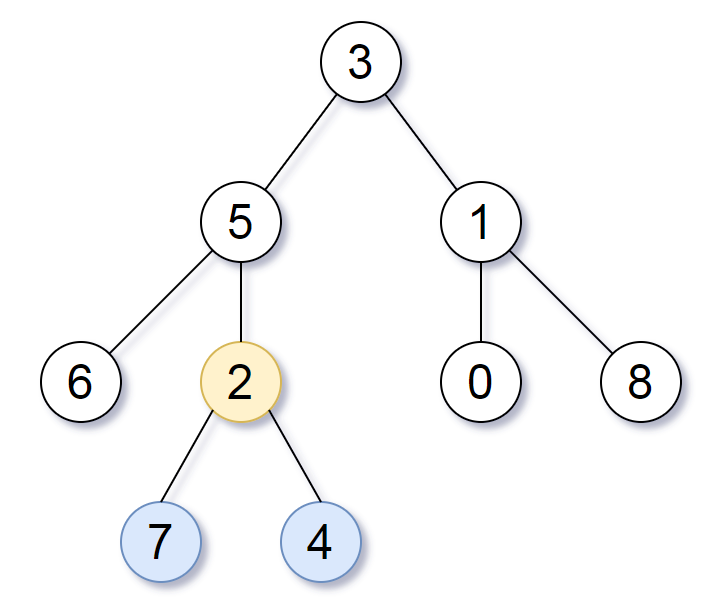Formatted question description: https://leetcode.ca/all/1123.html

# 1123. Lowest Common Ancestor of Deepest Leaves (Medium)

Given the root of a binary tree, return the lowest common ancestor of its deepest leaves.

Recall that:

• The node of a binary tree is a leaf if and only if it has no children
• The depth of the root of the tree is 0. if the depth of a node is d, the depth of each of its children is d + 1.
• The lowest common ancestor of a set S of nodes, is the node A with the largest depth such that every node in S is in the subtree with root A.

Note: This question is the same as 865: https://leetcode.com/problems/smallest-subtree-with-all-the-deepest-nodes/

Example 1:Input: root = [3,5,1,6,2,0,8,null,null,7,4]
Output: [2,7,4]
Explanation: We return the node with value 2, colored in yellow in the diagram.
The nodes coloured in blue are the deepest leaf-nodes of the tree.
Note that nodes 6, 0, and 8 are also leaf nodes, but the depth of them is 2, but the depth of nodes 7 and 4 is 3.

Example 2:

Input: root = 
Output: 
Explanation: The root is the deepest node in the tree, and it's the lca of itself.


Example 3:

Input: root = [0,1,3,null,2]
Output: 
Explanation: The deepest leaf node in the tree is 2, the lca of one node is itself.


Constraints:

• The number of nodes in the tree will be in the range [1, 1000].
• 0 <= Node.val <= 1000
• The values of the nodes in the tree are unique.

Related Topics:
Tree, Depth-first Search

Similar Questions:

## Solution 1.

// OJ: https://leetcode.com/problems/lowest-common-ancestor-of-deepest-leaves/

// Time: O(N)
// Space: O(H)
class Solution {
int maxDepth = -1, target = 0, cnt = 0;
void count(TreeNode *root, int d) {
if (!root) return;
if (d > maxDepth) {
target = 1;
maxDepth = d;
} else if (d == maxDepth) ++target;
count(root->left, d + 1);
count(root->right, d + 1);
}
TreeNode *find(TreeNode *root, int d) {
if (!root) return NULL;
int before = cnt;
auto left = find(root->left, d + 1);
if (left) return left;
auto right = find(root->right, d + 1);
if (right) return right;
if (d == maxDepth) ++cnt;
return before == 0 && cnt == target ? root : NULL;
}
public:
count(root, 0);
return find(root, 0);
}
};


## Solution 2.

The lowest ancester is the highest node whose left and right subtrees have the same height.

// OJ: https://leetcode.com/problems/lowest-common-ancestor-of-deepest-leaves/

// Time: O(N)
// Space: O(H)
class Solution {
pair<TreeNode*, int> dfs(TreeNode *root, int d = 0) { // latest node which has equal depth in left and right sub-trees; the corresponding height
if (!root) return {NULL, 0};
const auto &[left, ld] = dfs(root->left, d + 1);
const auto &[right, rd] = dfs(root->right, d + 1);
if (ld > rd) return {left, ld + 1};
else if (ld < rd) return{right, rd + 1};
return {root, ld + 1};
}
public:
return dfs(root).first;
}
};


Java

/**
* Definition for a binary tree node.
* public class TreeNode {
*     int val;
*     TreeNode left;
*     TreeNode right;
*     TreeNode(int x) { val = x; }
* }
*/
class Solution {
List<TreeNode> deepestLeaves = new ArrayList<TreeNode>();
Map<TreeNode, TreeNode> childParentMap = new HashMap<TreeNode, TreeNode>();
queue.offer(root);
while (!queue.isEmpty()) {
deepestLeaves.clear();
int size = queue.size();
for (int i = 0; i < size; i++) {
TreeNode node = queue.poll();
TreeNode left = node.left, right = node.right;
if (left == null && right == null)
if (left != null) {
childParentMap.put(left, node);
queue.offer(left);
}
if (right != null) {
childParentMap.put(right, node);
queue.offer(right);
}
}
}
if (deepestLeaves.size() == 1)
return deepestLeaves.get(0);
Set<TreeNode> set = new HashSet<TreeNode>();
for (TreeNode node : deepestLeaves) {
TreeNode parent = childParentMap.get(node);
}
while (set.size() > 1) {
List<TreeNode> list = new ArrayList<TreeNode>(set);
set.clear();
for (TreeNode node : list) {
TreeNode parent = childParentMap.get(node);